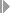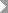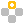Show TOC

###Results Analysis Methods#### Use

Which results analysis method you choose depends on your business requirements. A company will normally run different types of processing – and therefore use different methods of results analysis – simultaneously. Results analysis methods contain the rule for calculating the results analysis data.

#### Prerequisites

The standard system contains a number of different results analysis methods. Decide which results analysis method you want to use.

Choose a results analysis method in simplified Customizing for Product Cost by Sales Order underPeriod-End ClosingResults AnalysisValuation Method.

Decision Criteria

Capitalized Costs, Revenue in Excess of Billings, and Reserves

Legal requirements in different countries stipulate that unrealized profits can be capitalized or that they cannot be capitalized. This situation requires different balancing methods in the different countries.

• If you want to capitalize unrealized profits, use a results analysis method that can be used to create revenue in excess of billings.

• If you want to capitalize unrealized profits, use a results analysis method that can be used to create capitalized costs.

Some methods of creating capitalized costs and creating revenue in excess of billings also allow automatic creation of reserves for unrealized costs or reserves for imminent losses.

Milestone Billing / Milestone Delivery

If you want to use milestone billing or milestone delivery, use a results analysis method that allows creation of new capitalized costs in each period.

#### Features

For the system to be able to place the costs and revenues in relation to each other, a calculated percentage of completion (POC) must be generated. The percentage of completion can be calculated on the basis of the following data:

• R(p) planned revenues; C(p) planned costs; Q(p) planned quantity

• R(a) actual revenues; C(a) actual costs; Q(a) actual quantity

The system can calculate results analysis data using the percentage of completion. This data includes:

• C(z) capitalized costs (work in process)

• C(r) reserves for unrealized costs

• R(z) revenue in excess of billings

• R(r) revenue surplus

• R(PA) revenue affecting net income

• C(PA) costs affecting net income (cost of sales)

This results in the following formulas:

R(PA) = POC * R(p)

C(PA) = COS * C(p)

This has the following consequences:

• The revenue affecting net income is calculated by multiplying the percentage of completion by the planned revenue.

• The cost of sales is calculated by multiplying the percentage of completion by the planned costs.Example

End of the example.
• In the revenue-based results analysis method, the COS is calculated as follows:

• POC = R(a) / R(p)

• Therefore: R(PA) = R(a) / R(p) * R(p) = R(a)

• C(PA) = R(a) / R(p) * C(p)

• In the POC method, the COS is calculated as follows:

• POC = C(a) / C(p)

• Therefore: R(PA) = C(a) / C(p) * R(p)

• C(PA) = C(a) / C(p) * C(p) = C(a)

The following formula always holds:

• C(PA) < C(a) –-> C(z)

If the costs that affect net income are less than the actual costs, the system creates capitalized costs. The capitalized costs can be capitalized in the balance sheet through settlement to FI.

• C(PA) > C(a) --> C(r)

If the costs affecting net income are greater than the actual costs, the system creates reserves for unrealized costs. The reserves can be shown as a liability in the balance sheet through settlement to FI.

• R(PA) < R(a) --> R(r)

If the revenue affecting net income is less than the actual revenue, the system creates a revenue surplus. The revenue surplus is basically a reserve. The revenue surplus can be shown as a liability in the balance sheet through settlement to FI.

• R(PA) > R(a) --> R(z)

If the revenue affecting net income is greater than the actual revenue, the system creates revenue in excess of billings. The revenue in excess of billings can be capitalized in the balance sheet through settlement to FI.

#### Activities

Choose a results analysis method in Customizing for Product Cost by Sales Order underPeriod-End ClosingResults AnalysisDefine Valuation Method.

You can view the settings for the simplified results analysis methods in the Expert Mode of the valuation method.